# Expectation

## Notation

There's several different kinds of notation for the expectation that one might encounter.

First of all there is the different notations for the "E" part. It might be$\mathrm E$,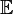$\mathbb E$,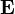$\mathbf E$, or something close to that. If the random variable is clear from context, it might be denoted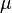$\mu$ or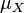$\mu_X$.

Second, there are various subscripts that can accompany the "E" part. The main ones are:

• Random variable as the subscript: e.g.$\mathbf E_X(X + Y)$. The idea here seems to be to specify that it is not the joint expectation, but the expectation over some specific random variable. 
• Distribution as the subscript: e.g.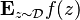$\mathbf E_{z \sim \mathcal D} f(z)$. I think the idea here is to de-emphasize the role of the random variable; we are saying something like "the expectation doesn't depend on the random variable itself, only its distribution, so we won't bother saying exactly what it is, only that it is sampled from this specific distribution".
• Parameter as subscript: in classical statistical inference, we are working with many probability measures (one for each value of the parameter$\theta$). So the subscript is used to specify which probability measure is being used to compute the expectation. e.g.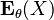$\mathbf E_\theta(X)$ means we are using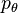$p_\theta$ or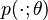$p(\cdot; \theta)$.

Questions: are some of the above subscripts actually equivalent? can all of them be written using the "bare" expectation notation (i.e. without the subscript)?